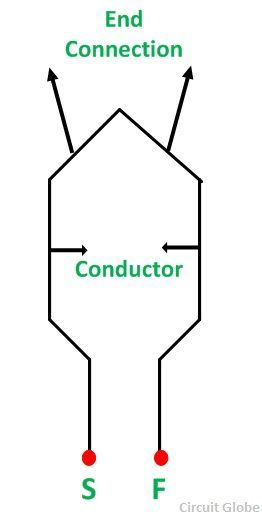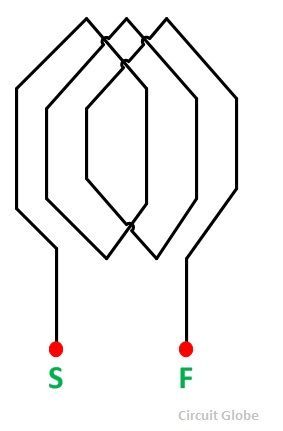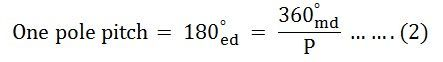# Armature Winding

Armature Winding is the winding, in which voltage is induced. Field Winding is the winding in which the main field flux is produced when the current through the winding is passed. Some of the basic terms related to the Armature Winding are defined as follows:

• Turn: A turn consists of two conductors connected to one end by an end connector.
• Coil: A coil is formed by connecting several turns in the series.
• Winding: A winding is formed by connecting several coils in series.

The figure of the turn is shown below.The figure of the coil is shown below.The figure of the winding is shown below.The beginning of the turn or coil is identified by the symbol (S) meaning Start, and the end of the turn or coil is represented by the symbol (F) meaning Finish.

The concept of electrical degree is very important in the study of the machine.

For a (P) pole machine, the electrical degree is defined as given below.Where,

?md is the mechanical degrees or an angular measure in space.

?ed is the electrical degrees or an angular measure in cycles.

The advantage of this notation is that the expressions written in terms of electrical angles apply to the machine with any number of poles.

The angular distance between the centers of two adjacent poles on a machine is known as pole pitch or pole span.The pole pitch is always 180 degrees electrical regardless of the number of poles in a machine.

The two sides of a coil are placed in two slots on the stator surface. The distance between the two sides of a coil is called the coil-pitch. If the coil pitch is one pole pitch, it is called the Full Pitch Coil. If the coil pitch is less than one pole pitch, the coil is called the Short Pitch or Fractional Pitch coil.

Also See: Coil Span Factor

Also See: Distribution Factor

### 2 thoughts on “Armature Winding”

1. Wow, I never knew that there was so much detail in coil machines. My dad was talking about them the other day, so I was curious enough to look it up. I think that my husband was wanting to buy one. I’ll have to ask him later.